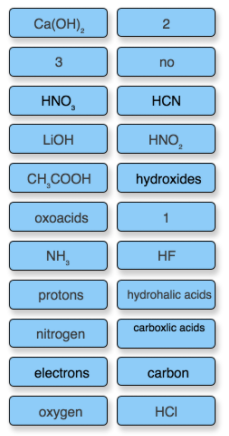# Problem: Complete the following sentences about strong and weak acids and bases. Strong acids are generally either hydrohalic acids (ex. _____) or oxoacids in which the number of oxygen atoms exceeds the number of ionizable _____ by 2 or more (ex. HNO3). Weak acids are usually one of the following: 1. hydrofluoric acid, _____, 2. acids in which the proton is not bonded to an oxygen atom or a halogen (ex. _____), 3. _____ where the number of oxygen atoms equals or only exceeds by 1 the number of ionizable _____ (ex. _____) or 4. _____ (ex. _____). Strong bases are _____ of group _____ metals (ex. _____) or larger group _____ metals (ex. _____), or oxides of the same metals. Weak bases are usually compounds containing a nitrogen atom with _____ bond(s) and 1 lone pair(s) (ex. _____).

###### FREE Expert Solution
96% (158 ratings)
###### Problem Details

Complete the following sentences about strong and weak acids and bases.

Strong acids are generally either hydrohalic acids (ex. _____) or oxoacids in which the number of oxygen atoms exceeds the number of ionizable _____ by 2 or more (ex. HNO3).

Weak acids are usually one of the following: 1. hydrofluoric acid, _____, 2. acids in which the proton is not bonded to an oxygen atom or a halogen (ex. _____), 3. _____ where the number of oxygen atoms equals or only exceeds by 1 the number of ionizable _____ (ex. _____) or 4. _____ (ex. _____).

Strong bases are _____ of group _____ metals (ex. _____) or larger group _____ metals (ex. _____), or oxides of the same metals.

Weak bases are usually compounds containing a nitrogen atom with _____ bond(s) and 1 lone pair(s) (ex. _____).What scientific concept do you need to know in order to solve this problem?

Our tutors have indicated that to solve this problem you will need to apply the Identifying Acids and Bases concept. You can view video lessons to learn Identifying Acids and Bases. Or if you need more Identifying Acids and Bases practice, you can also practice Identifying Acids and Bases practice problems.

What is the difficulty of this problem?

Our tutors rated the difficulty ofComplete the following sentences about strong and weak acids...as medium difficulty.

How long does this problem take to solve?

Our expert Chemistry tutor, Sabrina took 8 minutes and 29 seconds to solve this problem. You can follow their steps in the video explanation above.

What professor is this problem relevant for?

Based on our data, we think this problem is relevant for Professor Montano's class at UIC.